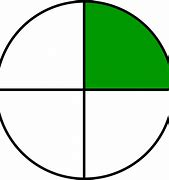# Maths Summer 6

## Maths

• Colour fractions of a circle.• Find half or quarter of a number
• To find half of a number means to share it into two equal parts.

1/2 of 8 = 4• To find a quarter of a number means to share it into four equal parts.

1/4 of 8 = 2• Commutative law-That addition can be done in any order, whereas subtraction has to start with the larger number. There is a worksheet and a dice game.• Can you read o’clock and half past times? Play the board game.1 o'clock               Half past 4